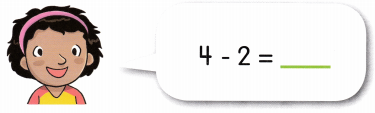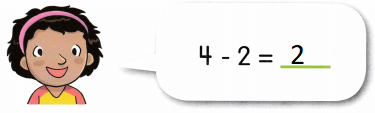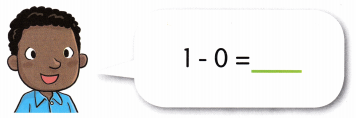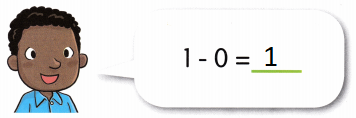# Math in Focus Kindergarten Chapter 18 Answer Key Subtraction Stories

Go through the Math in Focus Grade K Workbook Answer Key Chapter 18 Subtraction Stories to finish your assignments.

## Math in Focus Kindergarten Chapter 18 Answer Key Subtraction Stories

Lesson 1 Writing Subtraction Sentences and Representing Subtraction Stories

Count and write.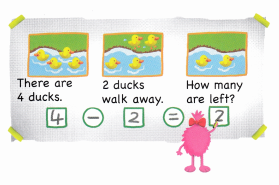Question 1.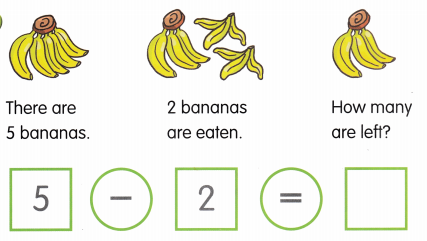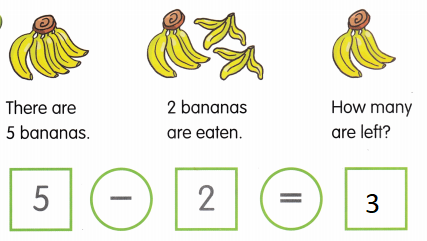Explanation:
The difference of 5 and 2 is 3
5 – 2 = 3

Question 2.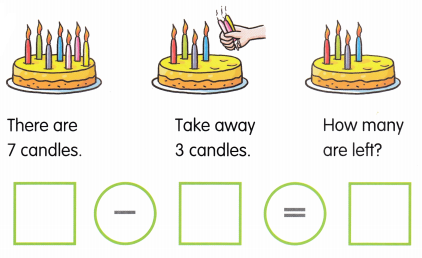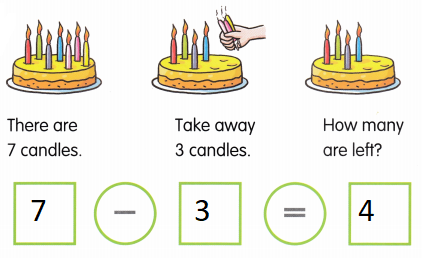Explanation:
The difference of 7 and 3 is 4
7 – 3 = 4

Question 3.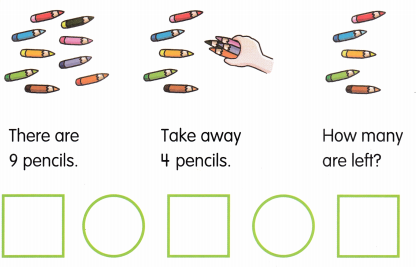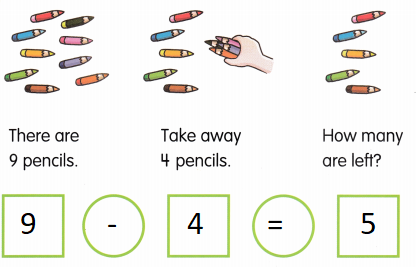Explanation:
The difference of 9 and 4 is 5
9 – 4 = 5

Count and write.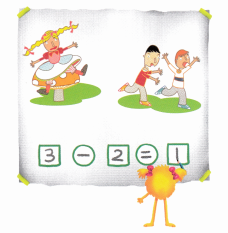Question 1.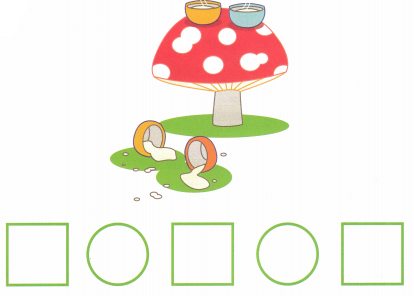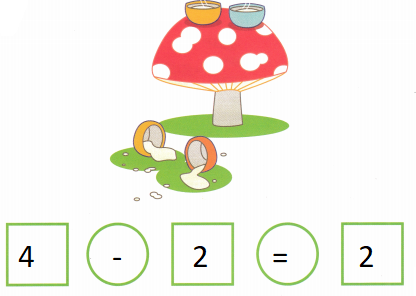Explanation:
The difference of 4 and 2 is 2
There are 4 bowls of soup out of that 2 fell down
4 – 2 = 2

Question 2.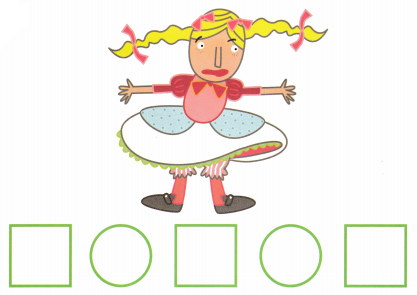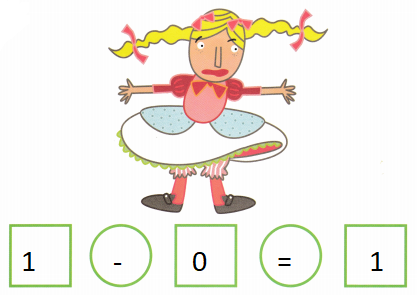Explanation:
The difference of 1 and 0
There is 1 girl and not going anywhere so, 1 – 0 = 1

Question 3.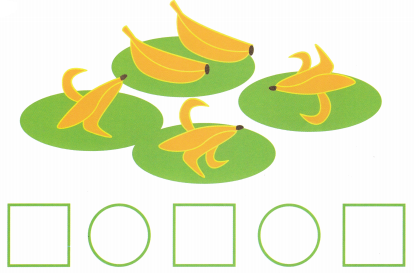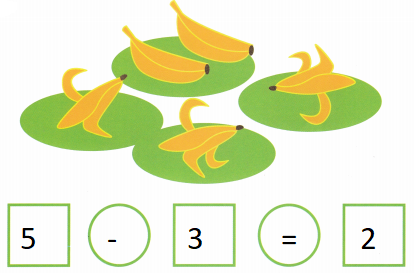Explanation:
The difference of 5 and 3 is 2
There are 5 bananas 3 were eaten
so, 2 are remaining
5 – 3 = 2

Question 4.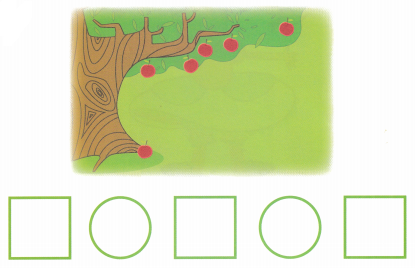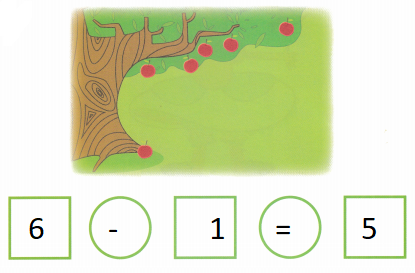Explanation:
The difference of 6 and 1 is 5
There are 6 apples and 1 fell down from the tree
6 – 1 = 5

Question 5.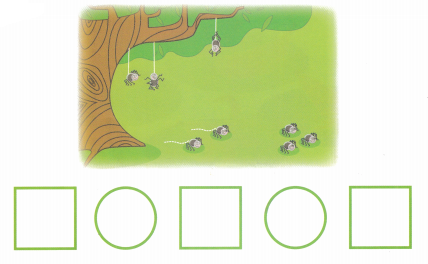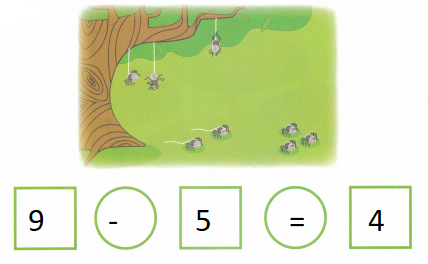Explanation:
The difference of 9 and 5 is 4
There are 9 spiders 5 fell down and 4 are still remaining on the tree
9 – 5 = 4

Lesson 2 Comparing Sets

How many more? Circle. Write the number sentence.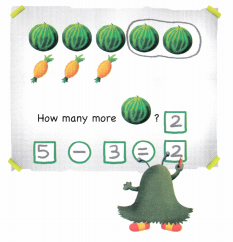Question 1.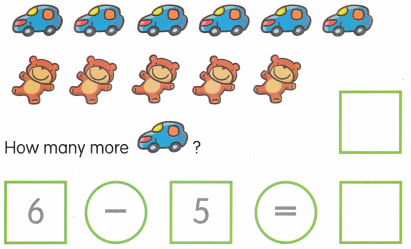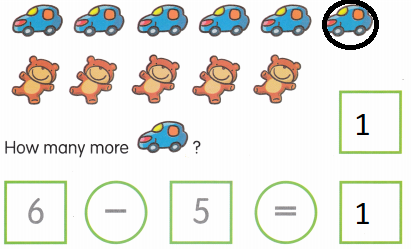Explanation:
The difference of 6 and 5 is 1
There are 6 cars and 5 teddy
6 – 5 = 1
so, there is 1 more car than teddy

Question 2.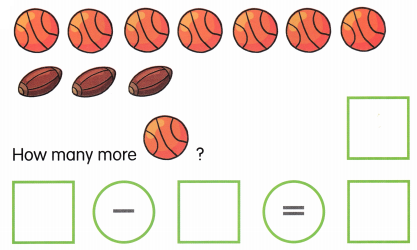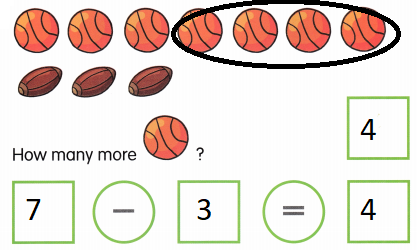Explanation:
The difference of 7 and 3 is 4
There are 7 basket balls and 3 American footballs
so, there are 4 more basket balls

Question 3.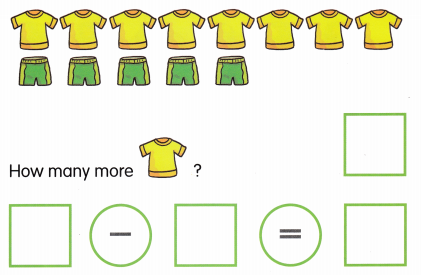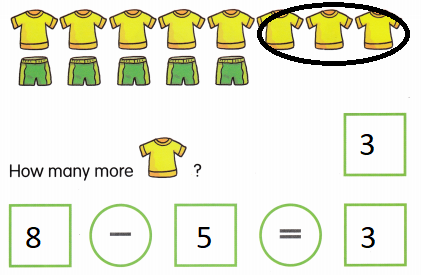Explanation:
The difference of 8 and 5 is 3
There 8 shirts out of it there are only 5 shorts
so there are 3 more shirts

Question 4.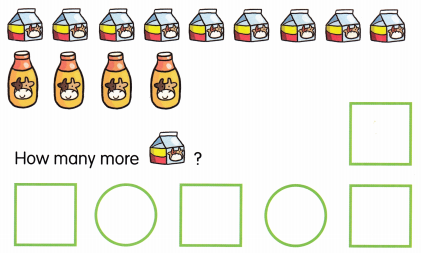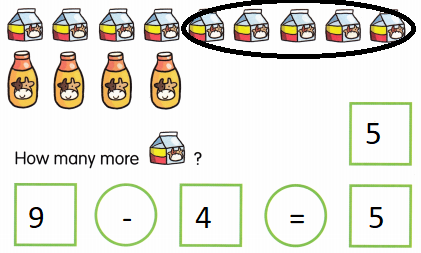Explanation:
The difference of 9 and 4 is 5
There are 9 milk packets and 4 milk bottles
so there are 5 more milk packets

Question 5.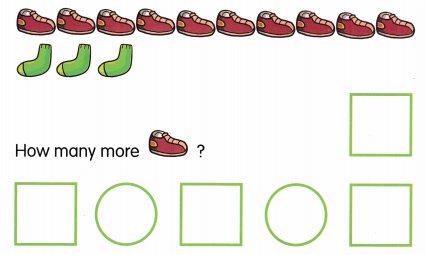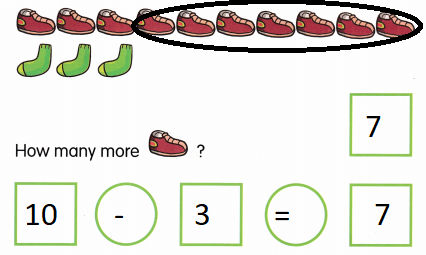Explanation:
The difference of 10 and 3 is 7
There are 10 shoes out of it only 3 pairs of socks are there
7 shoes are more

How many more? Write the number sentence.

Question 1.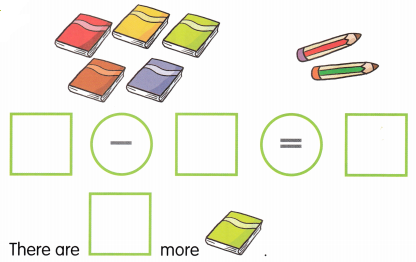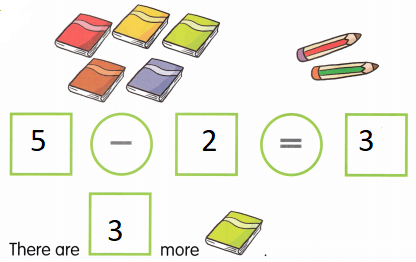Explanation:
The difference of 5 and 2 is 3
5 – 2 = 3
There are 3 more books than pencils

Question 2.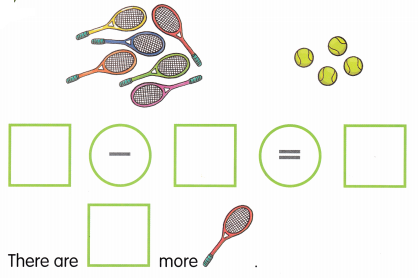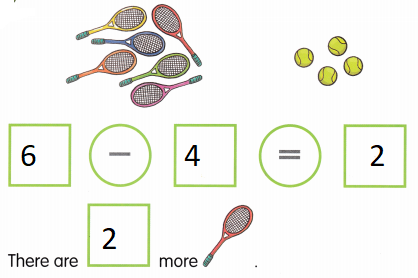Explanation:
The difference of 6 and 4 is 2
There are 2 more bats than balls

Question 3.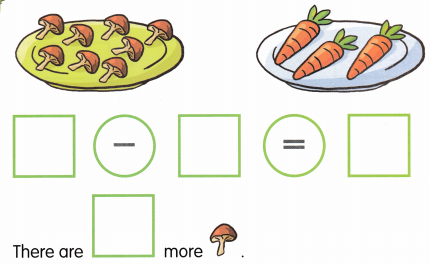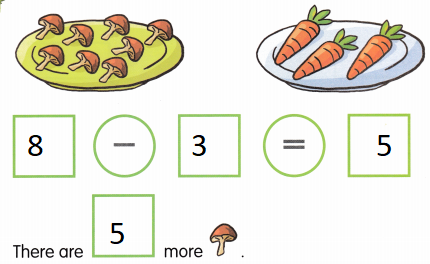Explanation:
The difference of 8 and 3 is 5
There are 5 more mushrooms than carrots

Question 4.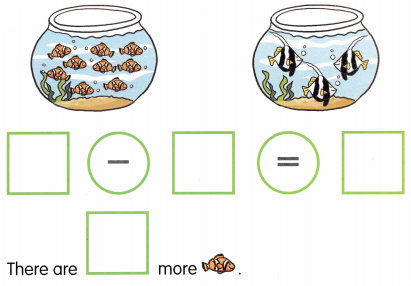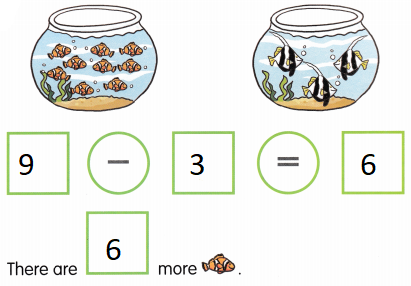Explanation:
The difference of 9 and 3 is 6
There are 6 more orange fishes than black fishes

Lesson 3 Subtraction Facts to 5

Match.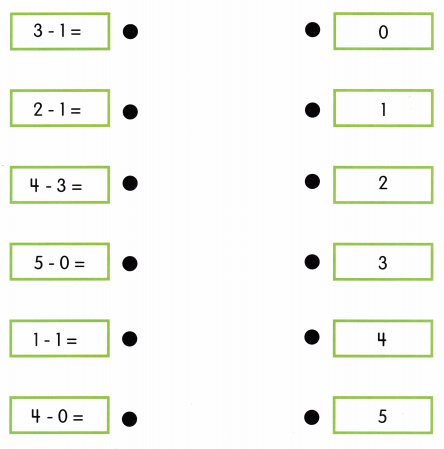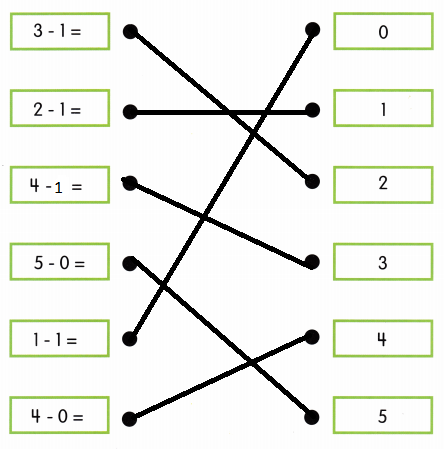Explanation:
The difference between the numbers are matched with the answers
3 – 1 = 2
2 – 1 = 1
4 – 1 = 3
5 – 0 = 5
1 – 1 = 0
4 – 0 = 4

Complete the number sentence.

Question 1.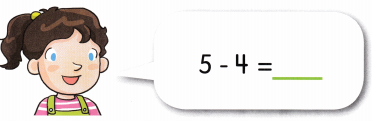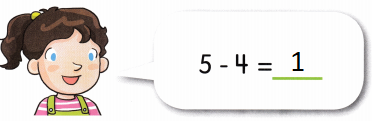Explanation:
The difference of 5 and 4 is 1
5 – 4 = 1

Question 2.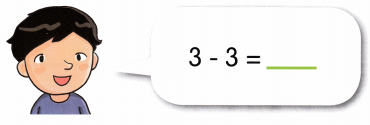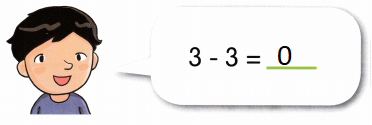Explanation:
The difference of 3 and 3 is 0
3 – 3 = 0

Question 3.